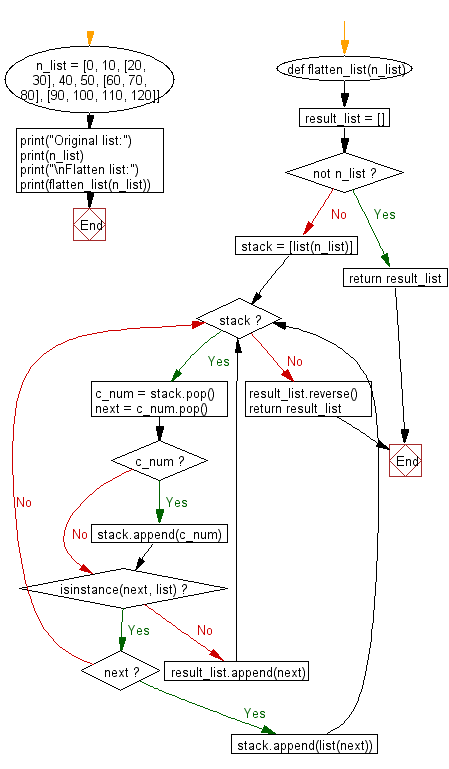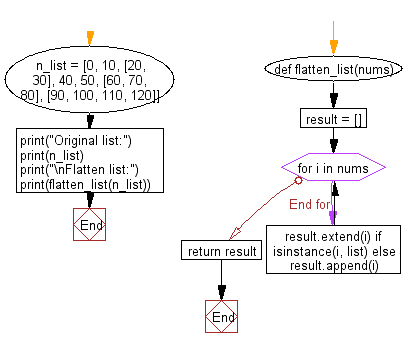﻿ Python: Flatten a given nested list structure - w3resource# Python: Flatten a given nested list structure

## Python List: Exercise - 72 with Solution

Write a Python program to flatten a given nested list structure.

Sample Solution-1:

Python Code:

``````def flatten_list(n_list):
result_list = []
if not n_list: return result_list
stack = [list(n_list)]
while stack:
c_num = stack.pop()
next = c_num.pop()
if c_num: stack.append(c_num)
if isinstance(next, list):
if next: stack.append(list(next))
else: result_list.append(next)
result_list.reverse()
return result_list
n_list = [0, 10, [20, 30], 40, 50, [60, 70, 80], [90, 100, 110, 120]]
print("Original list:")
print(n_list)
print("\nFlatten list:")
print(flatten_list(n_list))
```
```

Sample Output:

```Original list:
[0, 10, [20, 30], 40, 50, [60, 70, 80], [90, 100, 110, 120]]

Flatten list:
[0, 10, 20, 30, 40, 50, 60, 70, 80, 90, 100, 110, 120]
```

Flowchart:## Visualize Python code execution:

The following tool visualize what the computer is doing step-by-step as it executes the said program:

Sample Solution-2:

Loop over elements, use list.extend() if the element is a list, list.append() otherwise.

Python Code:

``````def flatten_list(nums):
result = []
for i in nums:
result.extend(i) if isinstance(i, list) else result.append(i)
return result
n_list = [0, 10, [20, 30], 40, 50, [60, 70, 80], [90, 100, 110, 120]]
print("Original list:")
print(n_list)
print("\nFlatten list:")
print(flatten_list(n_list))
```
```

Sample Output:

```Original list:
[0, 10, [20, 30], 40, 50, [60, 70, 80], [90, 100, 110, 120]]

Flatten list:
[0, 10, 20, 30, 40, 50, 60, 70, 80, 90, 100, 110, 120]
```

Flowchart:## Visualize Python code execution:

The following tool visualize what the computer is doing step-by-step as it executes the said program:

Python Code Editor:

Have another way to solve this solution? Contribute your code (and comments) through Disqus.

What is the difficulty level of this exercise?

Test your Programming skills with w3resource's quiz.

﻿

## Python: Tips of the Day

Difference between staticmethod and classmethod:

Maybe a bit of example code will help: Notice the difference in the call signatures of foo, class_foo and static_foo:

```class A(object):
def foo(self, x):
print "executing foo(%s, %s)" % (self, x)

@classmethod
def class_foo(cls, x):
print "executing class_foo(%s, %s)" % (cls, x)

@staticmethod
def static_foo(x):
print "executing static_foo(%s)" % x

a = A()
```

Below is the usual way an object instance calls a method. The object instance, a, is implicitly passed as the first argument.

```a.foo(1)
# executing foo(<__main__.A object at 0xb7dbef0c>,1)
```

With classmethods The class of the object instance is implicitly passed as the first argument instead of self.

```a.class_foo(1)
# executing class_foo(<class '__main__.A'>,1)
```

You can also call class_foo using the class. In fact, if you define something to be a classmethod, it is probably because you intend to call it from the class rather than from a class instance. A.foo(1) would have raised a TypeError, but A.class_foo(1) works just fine:

```A.class_foo(1)
# executing class_foo(<class '__main__.A'>,1)
```

One use people have found for class methods is to create inheritable alternative constructors.

With staticmethods neither self (the object instance) nor cls (the class) is implicitly passed as the first argument. They behave like plain functions except that you can call them from an instance or the class:

```a.static_foo(1)
# executing static_foo(1)

A.static_foo('hi')
# executing static_foo(hi)
```

Staticmethods are used to group functions which have some logical connection with a class to the class.

foo is just a function, but when you call a.foo you don't just get the function, you get a "partially applied" version of the function with the object instance a bound as the first argument to the function. foo expects 2 arguments, while a.foo only expects 1 argument.

a is bound to foo. That is what is meant by the term "bound" below:

```print(a.foo)
# <bound method A.foo of <__main__.A object at 0xb7d52f0c>>
```

With a.class_foo, a is not bound to class_foo, rather the class A is bound to class_foo.

```print(a.class_foo)
# <bound method type.class_foo of <class '__main__.A'>>
```

Here, with a staticmethod, even though it is a method, a.static_foo just returns a good 'ole function with no arguments bound. static_foo expects 1 argument, and a.static_foo expects 1 argument too.

```print(a.static_foo)
# <function static_foo at 0xb7d479cc>
```

And of course the same thing happens when you call static_foo with the class A instead.

```print(A.static_foo)
# <function static_foo at 0xb7d479cc>
```

Ref: https://bit.ly/2XFGOg6